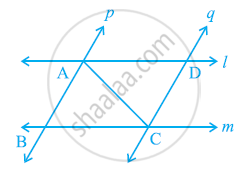Share

# l and m are two parallel lines intersected by another pair of parallel lines p and q (see the given figure). Show that ΔABC ≅ ΔCDA. - CBSE Class 9 - Mathematics

ConceptCriteria for Congruence of Triangles

#### Question

l and m are two parallel lines intersected by another pair of parallel lines p and q (see the given figure). Show that ΔABC ≅ ΔCDA.#### Solution

In ΔABC and ΔCDA,

∠BAC = ∠DCA (Alternate interior angles, as p || q)

AC = CA (Common)

∠BCA = ∠DAC (Alternate interior angles, as l || m)

∴ ΔABC ≅ ΔCDA (By ASA congruence rule)

Is there an error in this question or solution?

#### APPEARS IN

NCERT Solution for Mathematics Class 9 (2018 to Current)
Chapter 7: Triangles
Ex. 7.10 | Q: 4 | Page no. 119

#### Video TutorialsVIEW ALL 

Solution l and m are two parallel lines intersected by another pair of parallel lines p and q (see the given figure). Show that ΔABC ≅ ΔCDA. Concept: Criteria for Congruence of Triangles.
S